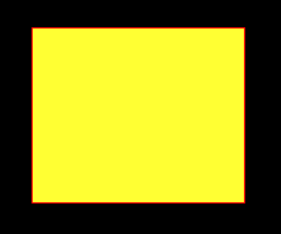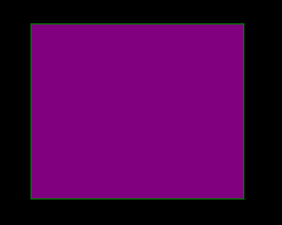# Python PIL – ImageDraw.Draw.rectangle()

## Python PIL | ImageDraw.Draw.rectangle()

PIL is the Python Imaging Library which provides the python interpreter with image editing capabilities. The `ImageDraw` module provide simple 2D graphics for Image objects. You can use this module to create new images, annotate or retouch existing images, and to generate graphics on the fly for web use.

`ImageDraw.Draw.rectangle()` Draws an rectangle.

Syntax: PIL.ImageDraw.Draw.rectangle(xy, fill=None, outline=None)
Parameters:

xy – Four points to define the bounding box. Sequence of either [(x0, y0), (x1, y1)] or [x0, y0, x1, y1]. The second point is just outside the drawn rectangle.
outline – Color to use for the outline.
fill – Color to use for the fill.

Returns: An Image object in rectangle shape.

 `  ` ` ` `# importing image object from PIL` `import` `math` `from` `PIL ``import` `Image, ImageDraw` ` ` `w, h ``=` `220``, ``190` `shape ``=` `[(``40``, ``40``), (w ``-` `10``, h ``-` `10``)]` ` ` `# creating new Image object` `img ``=` `Image.new(``"RGB"``, (w, h))` ` ` `# create rectangle image` `img1 ``=` `ImageDraw.Draw(img)  ` `img1.rectangle(shape, fill ``=``"# ffff33"``, outline ``=``"red"``)` `img.show()`

Output:Another Example: Here we use different colour for filling.

 `  ` ` ` `# importing image object from PIL` `import` `math` `from` `PIL ``import` `Image, ImageDraw` ` ` `w, h ``=` `220``, ``190` `shape ``=` `[(``40``, ``40``), (w ``-` `10``, h ``-` `10``)]` ` ` `# creating new Image object` `img ``=` `Image.new(``"RGB"``, (w, h))` ` ` `# create  rectangleimage` `img1 ``=` `ImageDraw.Draw(img)  ` `img1.rectangle(shape, fill ``=``"# 800080"``, outline ``=``"green"``)` `img.show()`

Output:Last Updated on March 1, 2022 by admin

## Action Chains in Selenium PythonAction Chains in Selenium Python

Action Chains in Selenium Python Selenium’s Python Module is built to perform automated testing with

## Opencv Python program for Face DetectionOpencv Python program for Face Detection

Opencv Python program for Face Detection The objective of the program given is to detect

## Deploy Python Flask App on HerokuDeploy Python Flask App on Heroku

Deploy Python Flask App on Heroku Flask is a web application framework written in Python.

## Desktop Notifier in PythonDesktop Notifier in Python

Desktop Notifier in Python This article demonstrates how to create a simple Desktop Notifier application using Python.

## Three-dimensional Plotting in Python using MatplotlibThree-dimensional Plotting in Python using Matplotlib

Three-dimensional Plotting in Python using Matplotlib Matplotlib was introduced keeping in mind, only two-dimensional plotting. But

## Python program to find the gcd of two numbersPython program to find the gcd of two numbers

Python program to find the gcd of two numbers Given two numbers. The task is

## Python – pass multiple arguments to map functionPython – pass multiple arguments to map function

Python – pass multiple arguments to map function The map() function is a built-in function